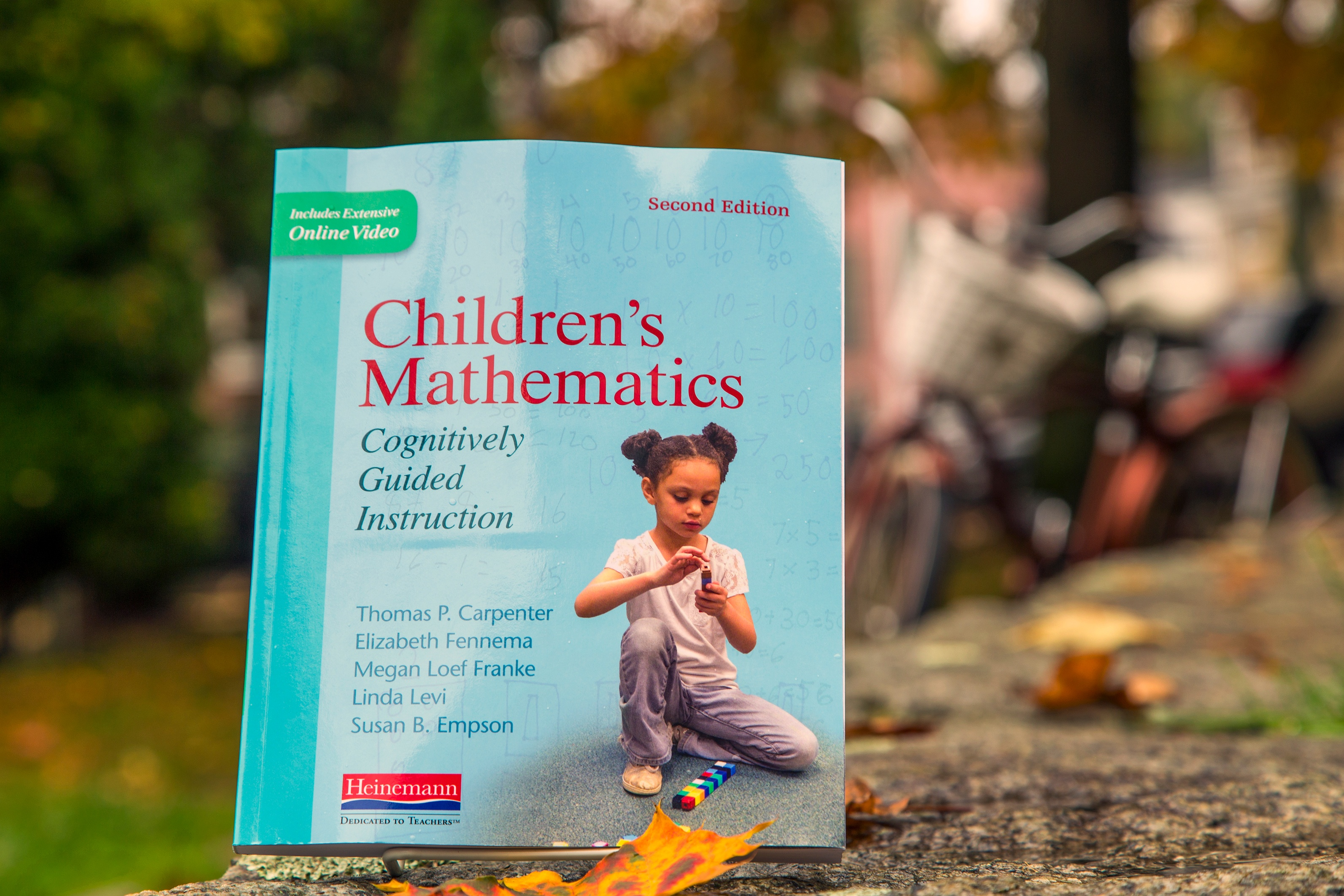<img height="1" width="1" alt="" style="display:none" src="https://www.facebook.com/tr?id=940171109376247&amp;ev=PageView&amp;noscript=1">

# Dedicated to TeachersIn anticipation of the new edition of Children’s Mathematics, Christopher Danielson writes about what he wishes all secondary teachers knew about Cognitively Guided Instruction (CGI).

Christopher taught in the Saint Paul Public Schools for six years, then earned a Ph.D. at Michigan State University. While a graduate assistant at Michigan State, he helped revise Connected Mathematics materials. Today he teaches in the math department at Normandale Community College, in Bloomington, MN, where he teaches college algebra, Calculus, and math content courses for future elementary teachers. He blogs at Overthinking My Teaching and Talking Math with Your Kids.

Why Every Math Teacher Should Know About Cognitively Guided Instruction
By Christopher Danielson

CGI, which is summarized in Children's Mathematics, tops my list of things I wish all secondary math teachers (and college math instructors) knew. There are two reasons—the content and the principle.

The Content
CGI lays out the ideas children bring to school about addition, subtraction, multiplication, division, and numbers more generally. It also identifies the four basic categories and eleven subcategories of addition and subtraction problems. All secondary math teachers should know this content.

Secondary math teachers teach algebra, and algebra is—in part—generalized arithmetic. If secondary teachers are fluently aware of the ideas children have about arithmetic, they are better positioned to help older students expand these ideas to accommodate learning algebra.

Here are two examples of how middle and high school instruction can be informed if the teacher knows how students think about basic arithmetic.

Subtraction

Many students, when they begin learning algebra, think of subtraction as taking away (the CGI term is separating). This idea makes it difficult to conceptualize subtracting negative numbers. How do you take away less than nothing? If this question isn’t answered, students revert to memorizing rules.

Teachers who know that taking away is how their students are likely to think about subtraction and also know that comparing is another powerful way of thinking about subtraction can help their students see 3 – -2 as a matter of the distance between 3 and -2 on the number line: how much bigger is 3 than -2? The calculation can be made by comparing the numbers.

Multiplication

Sometimes the expression 3x means I have 3 groups of an unknown size and sometimes it means I have an unknown number of groups of 3. To an algebra teacher, these are equivalent concepts, because an algebra teacher has an abstract understanding of multiplication. We want students to develop this abstract understanding too, to understand multiplication as an operation, so that they can use it to study and understand exponentiation, calculus, and modern algebra. But not all students achieve this.

If all algebra teachers know that their students often think very literally about multiplication and find literal and operational interpretations very different, they can design their instruction to address this misconception.

Maybe some students are better served if they write x3 instead of 3x when they first try to represent an unknown number of groups of 3. The point is that knowing how students think about these ideas helps a teacher make instructional decisions and see new instructional possibilities.

The principle
Students arrive in our classrooms with ideas; they are not blank slates. Cognitively Guided Instruction is based on the ideas students already have. This principle should inform all mathematics instruction: let’s teach algebra based on the ideas students have about variables, geometry based on their ideas about shapes, and so on. Children's Mathematics shows us what this looks like in the classroom.